PHY_10_35_V2_DM_E&G fields
Оценка 4.7

# PHY_10_35_V2_DM_E&G fields

Оценка 4.7
docx
07.05.2020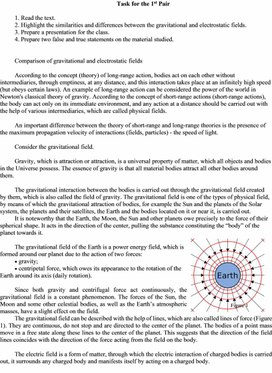PHY_10_35_V2_DM_E&G fields.docx

2. Highlight the similarities and differences between the gravitational and electrostatic fields.

3. Prepare a presentation for the class.

4. Prepare two false and true statements on the material studied.

Comparison of gravitational and electrostatic fields

According to the concept (theory) of long-range action, bodies act on each other without intermediaries, through emptiness, at any distance, and this interaction takes place at an infinitely high speed (but obeys certain laws). An example of long-range action can be considered the power of the world in Newton's classical theory of gravity. According to the concept of short-range actions (short-range actions), the body can act only on its immediate environment, and any action at a distance should be carried out with the help of various intermediaries, which are called physical fields.

An important difference between the theory of short-range and long-range theories is the presence of the maximum propagation velocity of interactions (fields, particles) - the speed of light.

Consider the gravitational field.

Gravity, which is attraction or attraction, is a universal property of matter, which all objects and bodies in the Universe possess. The essence of gravity is that all material bodies attract all other bodies around them.

The gravitational interaction between the bodies is carried out through the gravitational field created by them, which is also called the field of gravity. The gravitational field is one of the types of physical field, by means of which the gravitational attraction of bodies, for example the Sun and the planets of the Solar system, the planets and their satellites, the Earth and the bodies located on it or near it, is carried out.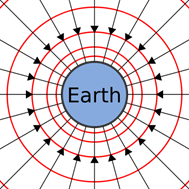It is noteworthy that the Earth, the Moon, the Sun and other planets owe precisely to the force of their spherical shape. It acts in the direction of the center, pulling the substance constituting the “body” of the planet towards it.

The gravitational field of the Earth is a power energy field, which is formed around our planet due to the action of two forces:

·  gravity;

·  centripetal force, which owes its appearance to the rotation of the Earth around its axis (daily rotation).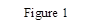Since both gravity and centrifugal force act continuously, the gravitational field is a constant phenomenon. The forces of the Sun, the Moon and some other celestial bodies, as well as the Earth’s atmospheric masses, have a slight effect on the field.

The gravitational field can be described with the help of lines, which are also called lines of force (Figure 1). They are continuous, do not stop and are directed to the center of the planet. The bodies of a point mass move in a free state along these lines to the center of the planet. This suggests that the direction of the field lines coincides with the direction of the force acting from the field on the body.

The electric field is a form of matter, through which the electric interaction of charged bodies is carried out, it surrounds any charged body and manifests itself by acting on a charged body.

Based on this definition, the principle of interaction between two charged bodies can be clarified: an electric field forms around one charged body and its own electric field forms around the other body, the fields are in contact, and it is through this intermediate link that the electric interaction between charged bodies is transmitted.

Since the electric field is one of the types of matter, it has certain properties:

– created around a resting electric charge;

– determined by the effect on another electric charge.

Note that the concept of an electric field is global with respect to all electrical interactions and all electrical interactions are accompanied by the formation of an electric field.

In the following, we will dwell in greater detail on the fact that the electric field is a component of a more complex type of matter of the electromagnetic field. But now we can already note that all the matter of the world is divided into two types: matter and field.

Such a field surrounds each electric charge and acts on other charges with some force. Therefore, the electric field is a kind of force field.

Graphically, the electric field is depicted using arrows.

A line of force (arrow) is such a line, the tangent to which at each point coincides with the direction of the intensity vector at that point.

The lines of force begin at positive charges or at infinity and end in negative ones, or go off to infinity. They never intersect or touch each other.

The lines of force indicate the direction of the force acting on a positively charged particle from the side of the electric field.

In general, these lines are shaped like curves. But they can also be straight lines if the field of a single point charge is described (Figure 2).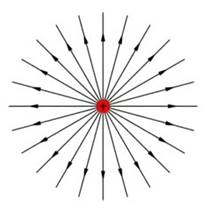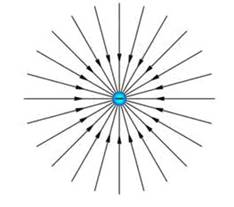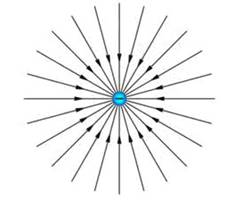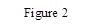Think and complete tasks in a pair on this sheet.

1.                       Describe what the lines of force means

(i)                     Gravitational field …………….

(ii)                   Electric field ………………

2.                       The charged metal sphere is in an isolated space. Write one similarity and one difference between the gravitational and electric fields around the sphere:

(i)                     Similarity ……………….

(ii)                   Difference ……………….

3.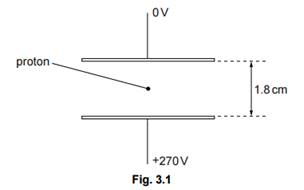Two horizontal metal plates are located at a distance of 1.8 cm from each other in a vacuum. The potential difference between them is 270 V, as shown in the figure.

The proton is in the space between the plates. Explain qualitatively and quantitatively why the gravitational field is not taken into account when a proton moves in between the plates.

2. Highlight the similarities and differences between the gravitational and electrostatic fields.

3. Prepare a presentation for the class.

4. Prepare two false and true statements on the material studied.

Uniform gravitational and electrostatic field

Uniform field is called the field of tension of which field is the same in all locations. This field lines, which perfectly parallel to each other, as well as their density per unit area will also be constant.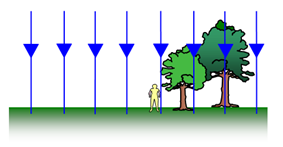Using power lines can be quantified the electric field intensity. For this density, or density, power lines selected is proportional to the tension vector module. The density of field lines is defined as the number of lines contained within a single surface in a direction perpendicular to this surface.

The gravitational field of the Earth or another planet can be considered homogeneous near its surface. For example, compared to the size of small bodies, such as automobiles, airplanes and balloons.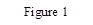For small heights at this scale (several tens of kilometers), the field strength does not change enough to be noticeable.  Again, the field lines are directed to the center of the Earth, and a loose body near the surface of the Earth will fall along these lines (Figure 1).

We already know that the space around the body charged electrical field exists (one type of matter), having a stock of electric energy. This energy manifests itself in the form of electric forces acting on the field of charged particles.

Conventionally, the electric field is portrayed in the form of electrical power lines, which show the direction of electric force generated by the field. Accepted direct power line in the wrong direction, which would be moved into the field of positively charged particle.

Field created one infinite charged plane and two flat unlikely charged parallel plates, called uniform. Graphically, this field shows the parallel straight lines with the same distance between lines (Figure 2).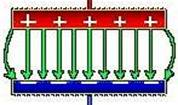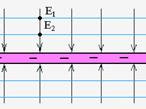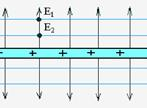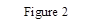Gravitational and electrostatic field operate on moving the body. If the body has a initial time speed aimed perpendicular to the field lines, its trajectory changes under the influence of force fields (Figure 3). An important difference in the gravitational field from electric is that because body weight is a positive value, then the body will deviate from the original direction of motion along the field lines. In the case of electric charge, this will depend on charge sign (Figure 3).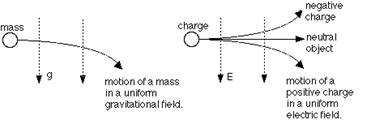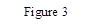You can see the movement on the parabola, where electrons move in a uniform field with an initial velocity perpendicular to the field. Then from the second law of Newton = eE / m.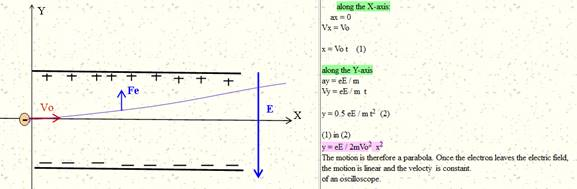This movement of electrons in a cathode ray tube.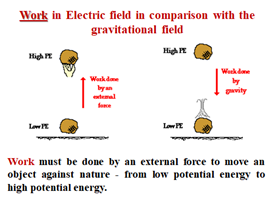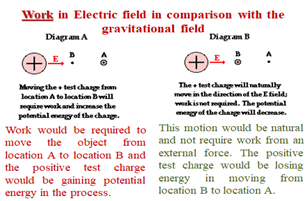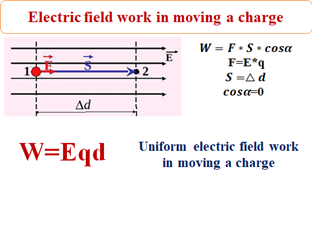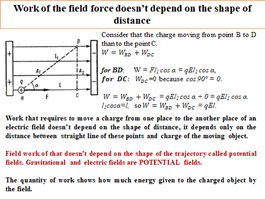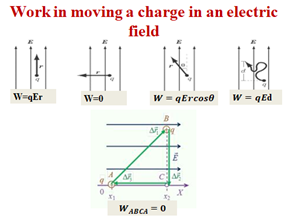Think and complete tasks in a pair on this sheet.

1.                       Describe what the lines of force means

(i)                     Gravitational field …………….

(ii)                   Electric field ………………

2.                       The charged metal sphere is in an isolated space. Write one similarity and one difference between the gravitational and electric fields around the sphere:

(i)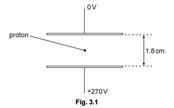Similarity ……………….

(ii)                   Difference ……………….

3.                        Two horizontal metal plates are located at a distance of 1.8 cm from each other in a vacuum. The potential difference between them is 270 V, as shown in the figure.

The proton is in the space between the plates. Explain qualitatively and quantitatively why the gravitational field is not taken into account when a proton moves in between the plates.

2. Highlight the similarities and differences between the gravitational and electrostatic fields.

3. Prepare a presentation for the class.

4. Prepare two false and true statements on the material studied.

Due to gravitational gravitation people and animals can safely move around the Earth, rivers, seas and oceans-remain within their shores, and the air-do not fly across the endless expanse of space and form an atmosphere of our planet.

A fair question arises: If all the objects have gravity, why the Earth attracts people and animals, and not vice versa? Firstly, we attract to the Earth, just as compared to its force of attraction our gravity is negligible. Secondly, the force of gravity is directly proportional to the mass of the body: the lower the weight, the lower its gravitational force.

The second indicator is dependent on the power of attraction is the distance between objects: the greater the distance, the less the effect of gravity. Including through this planet are moving in their orbits, rather than falling on each other.

The law of gravity was formulated by Isaac Newton (1643−1727) and published in the year 1687. Under this law, two point body attract each other with a force that is directly proportional to the masses m1 and m2 of the bodies and inversely proportional to the square of the distance between them: F=Gm1m2/r2.

Here r − distance between these bodies, G − gravitational constant whose value found experimentally, is G=6,67×10−11m3kgs2.

The strength of the gravitational attraction is a central force, i.e. along a straight line, passing through the centres of interacting bodies (Figure 1).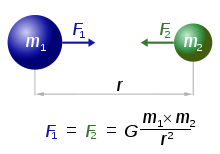Force of gravity may not be pushing. In addition, gravitational interaction cannot be loosen or eliminate any screen.

It can be applied:

a) for material points;

b) for bodies having the form of a ball;

c) for a ball of large radius interacting with bodies whose dimensions are much smaller than the size of the ball.

Coulomb's law is one of the basic laws of electrostatics. It determines the magnitude and direction of the interaction force between two fixed point charges.

Under the point charge understand a charged body, the size of which is much less than the distance of its possible impact on other bodies. In this case, neither the shape nor the size of the charged bodies affect practically the interaction between them.

In 1785, the law was established by O. Coulomb using special torsional weights. Experiments Coulomb allowed to establish a law, strikingly reminiscent of the law of the world.

The force of interaction of two point stationary charged bodies in vacuum is directly proportional to the product of charge modules and inversely proportional to the square of the distance between them.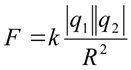Where |q1| and |q2| — modules of charges; r — the distance between them; k — the proportionality constant, depending on the choice of unit system  k = 9 · 109N·m2/kg2.

The interaction force is directed along a straight line connecting the charges, the like charges repel each other, while opposite charges attract each other (Figure 2).

The interaction force between the charges also depends on the medium between the charged bodies.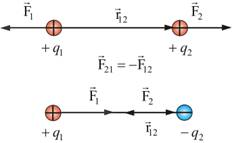In the air, the force of interaction hardly differs from that in vacuum. Coulomb's law expresses the interaction of charges in a vacuum.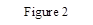It is important to note that in order for the law to be true, the following are necessary:

1.                      the accuracy of the charges - that is, the distance between charged bodies is much larger than their size;

2.                      their immobility. Otherwise, additional effects should be taken into account: the arising magnetic field of a moving charge and the corresponding additional Lorentz force acting on another moving charge;

3.                      interaction in a vacuum.

However, with some adjustments, the law is also valid for interactions of charges in the medium and for moving charges.

Think and complete tasks in a pair on this sheet.

1.                       Describe what the lines of force means

(i)                     Gravitational field …………….

(ii)                   Electric field ………………

2.                       The charged metal sphere is in an isolated space. Write one similarity and one difference between the gravitational and electric fields around the sphere:

(i)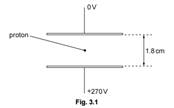Similarity ……………….

(ii)                   Difference ……………….

3.                        Two horizontal metal plates are located at a distance of 1.8 cm from each other in a vacuum. The potential difference between them is 270 V, as shown in the figure.

The proton is in the space between the plates. Explain qualitatively and quantitatively why the gravitational field is not taken into account when a proton moves in between the plates.

2. Highlight the similarities and differences between the gravitational and electrostatic fields.

3. Prepare a presentation for the class.

4. Prepare two false and true statements on the material studied.

Any body creates a gravitational field around itself. The strength characteristic of a gravitational field is its intensity.

Let us need at some point in space to characterize the gravitational field created by a certain body of mass M. Place the “test” point body of mass m1 in the point of space (field) of interest to us and measure the force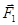from which the field will act on it. If we change the mass of the “test” body: m1 → m2 → …→ mn, the experience shows that the force acting on him will change: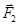… →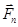, However, the ratio of the force acting on it to its mass will not depend on the mass of the “test” body.:/m1=/m2 = … =/mn . Consequently, this relationship already depends only on the field and, therefore, is a characteristic of the field at a given point in space and is called the gravitational field strength at a given location: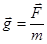(2)

Tension – vector the characteristic of the field, she directed (as m > 0) there, where the force of attraction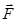– i.e. in the direction of body weight М - the body, which generates this field. Numerically equal tension force, acting on "trial" acupressure body with a single weight placed in the given point of the field. The dimension of the tension field matches the dimensionality accelerate-international system of units (SI) is N/kg = m/s2.

The tension of the gravitational field is the same as the largest, and units with the acceleration of free fall, although its physical meaning is totally different physical quantities. While the field strength characterizes the State of space at this point, power and Acceleration only appear when the trial is at this point the body.

If we know the tension g (r) of the gravitational field at any point in space r, it follows from the definition (2), we can calculate the force, which will operate on the spot the body of mass m, placed in this field point:

F = mg

Consider the gravitational field created by point weight, m. It is obvious that it has spherical symmetry – vector tension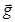at any point is directed to the mass m, creates a field and is equal in magnitude as the law (1)

g(r) = F/m = GM/r2 (3)

- depends only on the distance r to the source fields.

How to calculate the tension of the gravitational field produced by a pinhole body and multiple bodies? Experience has shown that gravitational fields satisfy the principle of superposition. He states that the gravitational field generated by any mass does not depend on the existence of other mass. Field strength generated by several bodies, is equal to the vector sum of the tensions created by these bodies fields individually.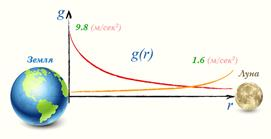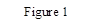From the graph of a function g = g (r) can be clearly seen that the tension of the gravitational field g tends to zero, when the distance r tends to infinity. Therefore, the approval of a type of Sputnik left the gravitational field of the Earth is incorrect (Figure 1).

The gravitational fields of celestial bodies overlap. If we move along the straight line connecting the centers of the Earth and the Moon, then, starting from a certain place, the intensity of the gravitational field of the Moon will prevail.

Electric field strength. The quantitative characteristic of the force action of the electric field on charged bodies is the vector quantity E, called the electric field strength.

E = F / q пр.

It is determined by the ratio of the force F acting on the part of the field on pinpoint test charge qпр, placed in the considered point fields to this charge.

The concept of "test charge" suggests that this charge is not involved in the creation of an electric field and so small that it does not distort it, i.e. it does not cause the redistribution of the charge space, creating a field in question. In SI, the unit of tension serves 1/m, which is equivalent to 1 N/kg.

The field strength of a point charge. Using the Coulomb law, you can find an expression for the electric field intensity produced by the point charge q in a homogeneous isotropic Wednesday at a distance r from the charge: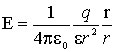(1.2)

In this formula, r is the radius vector connecting the charges qи qпр. (1.2) that electric field strength (E) of a point charge qvo all points of the field is directed radially from the charge at q > 0 and to charge at q < 0.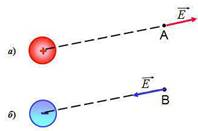Electrical field strength the magnitude of the vector. If the field created a positive charge, it is directed from the considered point "from the charge", otherwise "the charge" (Figure 2).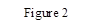Principle of superposition of fields: field strength created by charges is equal to the geometric sum of tensions generated by each field. I.e. the tensions add up geometrically: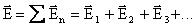(It is a fact.)

Think and complete tasks in a pair on this sheet.

1.                       Describe what the lines of force means

(i)                     Gravitational field …………….

(ii)                   Electric field ………………

2.                       The charged metal sphere is in an isolated space. Write one similarity and one difference between the gravitational and electric fields around the sphere:

(i)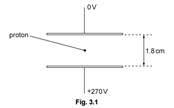Similarity ……………….

(ii)                   Difference ……………….

3.                        Two horizontal metal plates are located at a distance of 1.8 cm from each other in a vacuum. The potential difference between them is 270 V, as shown in the figure.

The proton is in the space between the plates. Explain qualitatively and quantitatively why the gravitational field is not taken into account when a proton moves in between the plates.

2. Highlight the similarities and differences between the gravitational and electrostatic fields.

3. Prepare a presentation for the class.

4. Prepare two false and true statements on the material studied.

 Gravity work near the surface of the earth The work force uniform electric field Gravitational field near the surface of the Earth and the electrostatic field of two charged plates It is a uniform field. Since from the side of the field a force acts on the body / charge that causes the body to move, the field does work. W= (mgh1- mgh2) W= (qEd1- qEd2) From the obtained formulas it follows that the work of the forces of the gravitational / homogeneous electrostatic field does not depend on the shape of the trajectory and it is zero along a closed trajectory. A field whose work along a closed trajectory is zero is called potential. Gravitational / electrostatic uniform field - a potential field. The work is associated with a change in energy as follows W = - (Ep2 – Ep1) Then the potential energy of a body raised above the Earth is calculated by the formula Ep=mgh. In the case of an electrostatic uniform field Wp =qEd The gravitational / electrostatic field, besides the power characteristic, also has an energy characteristic - potential. Potential is a scalar quantity. The potential allows you to determine what potential energy the body / charge possesses at a given point of the field. The physical meaning of the potential: the potential of the field at a given point is numerically equal to the work of moving a single positive charge from a given point to infinity. Ep=mφ. Wp =qφ The potential of the gravitational field can take values greater than or equal to zero. The potential at any point of the field created by a positive charge q is positive, and the field created by a negative charge is negative:если q> 0, then φ > 0; if q< 0, then φ < 0. The gravitational / electrostatic uniform field is usually described using field lines and equipotential surfaces. Equipotential surface is a locus of points with equal potential. Knowing how the potential of a field created by a spherical body / point charge (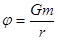;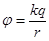), you can build equipotential surfaces. The potential, as well as the potential energy of a charge, depends on the choice of the reference system (zero level). In the technique for the potential zero choose the potential of the surface of the Earth or a conductor connected to the ground. This conductor is called grounded. In physics, the origin (zero level) of the potential (and potential energy) is taken as any point that is infinitely distant from the bodies / charges that create the field. Equipotential surfaces of the gravitational field are shown with red lines.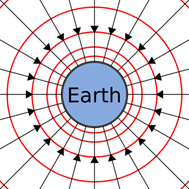Equipotential Surfaces of a Point Charged Field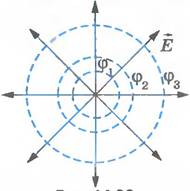W= m(φ1- φ2) W= q(φ1- φ2) The principle of superposition: the potential φ of the field created by the system of bodies / charges at some point in space is equal to the algebraic sum of the potentials created at this point by each charge separately:  φ=φ1+φ2+φ3+...=∑ni=1φi. Potential difference — this is a scalar physical quantity, numerically equal to the ratio of the work of the field forces in charge transfer between given points of the field to this charge. In SI, the unit of potential difference is volt (V).   1 V - the potential difference between two points of the electrostatic field, when moving between them a charge of 1 C, the field forces perform work in 1 J. The potential difference in contrast to the potential does not depend on the choice of the zero point. The potential difference φ1 - φ2 is often called the electrical voltage between given field points and is denoted by U:  U=φ1−φ2. Voltage between two points of the field is determined by the work of the forces of this field to move a charge in 1 C from one point to another.

Think and complete tasks in a pair on this sheet.

1.                       Describe what the lines of force means

(i)                     Gravitational field …………….

(ii)                   Electric field ………………

2.                       The charged metal sphere is in an isolated space. Write one similarity and one difference between the gravitational and electric fields around the sphere:

(i)Similarity ……………….

(ii)                   Difference ……………….

3.                        Two horizontal metal plates are located at a distance of 1.8 cm from each other in a vacuum. The potential difference between them is 270 V, as shown in the figure.

The proton is in the space between the plates. Explain qualitatively and quantitatively why the gravitational field is not taken into account when a proton moves in between the plates.

Cards to divide students into groups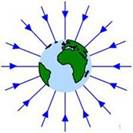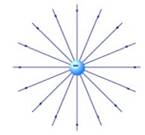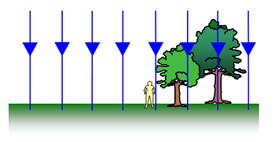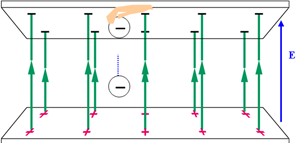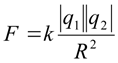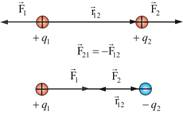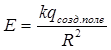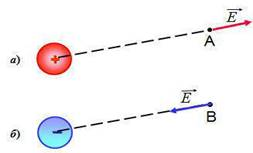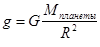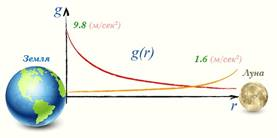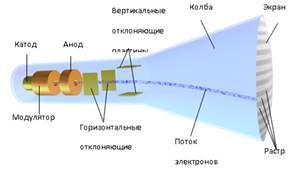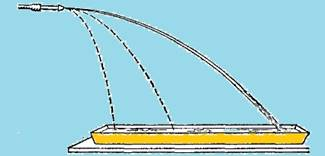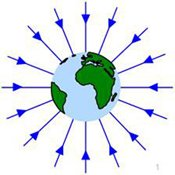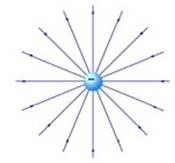Скачано с www.znanio.ru

## Based on this definition, the principle of interaction between two charged bodies can be clarified: an electric field forms around one charged body and its…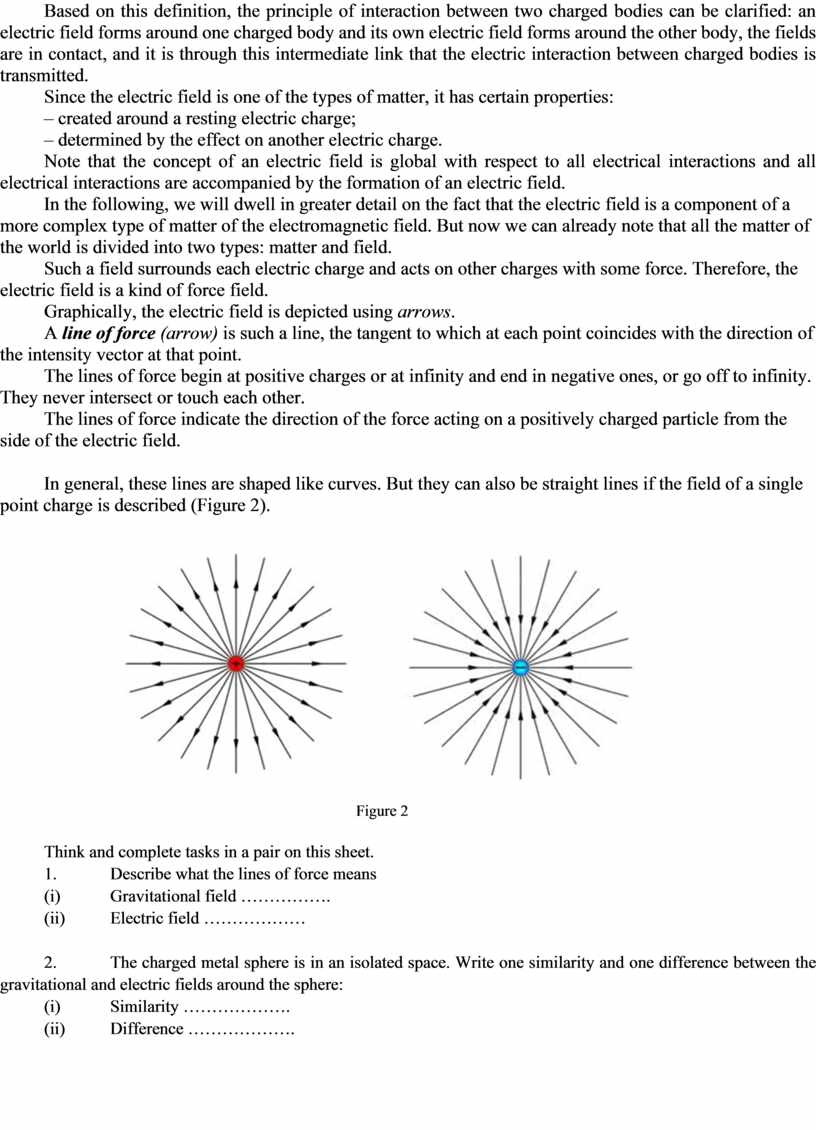## Two horizontal metal plates are located at a distance of 1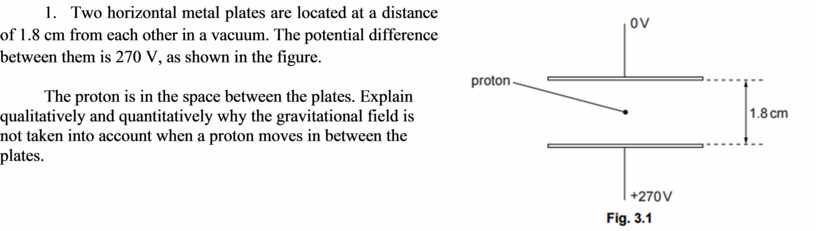## You can see the movement on the parabola, where electrons move in a uniform field with an initial velocity perpendicular to the field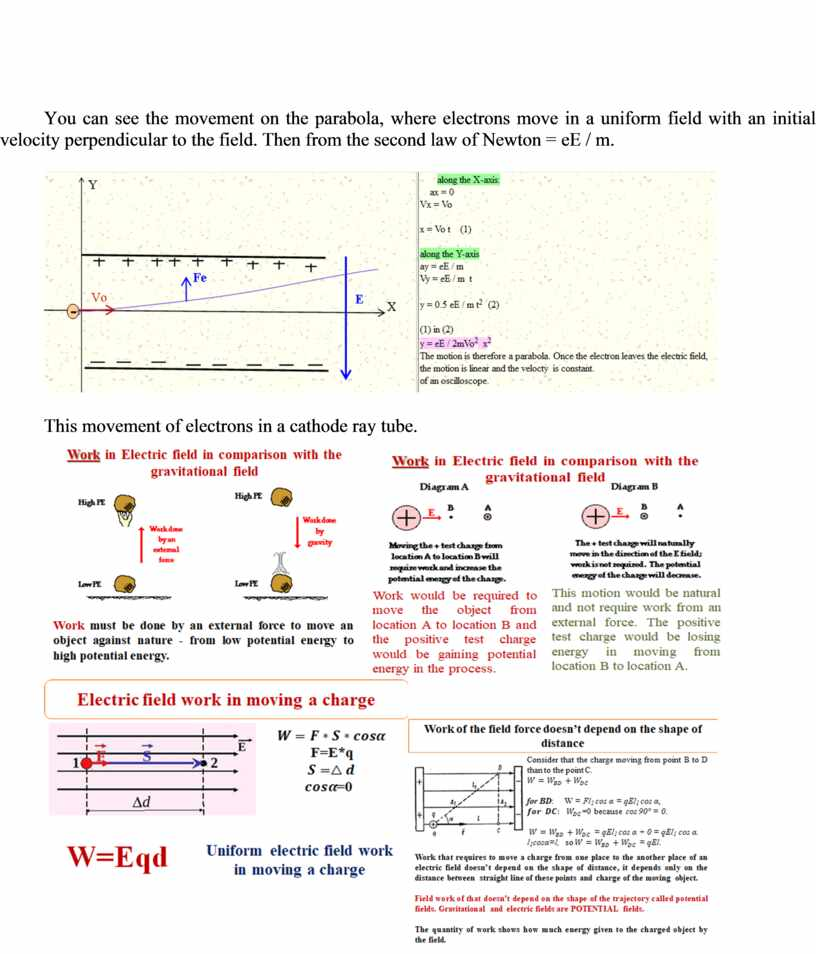## The interaction force between the charges also depends on the medium between the charged bodies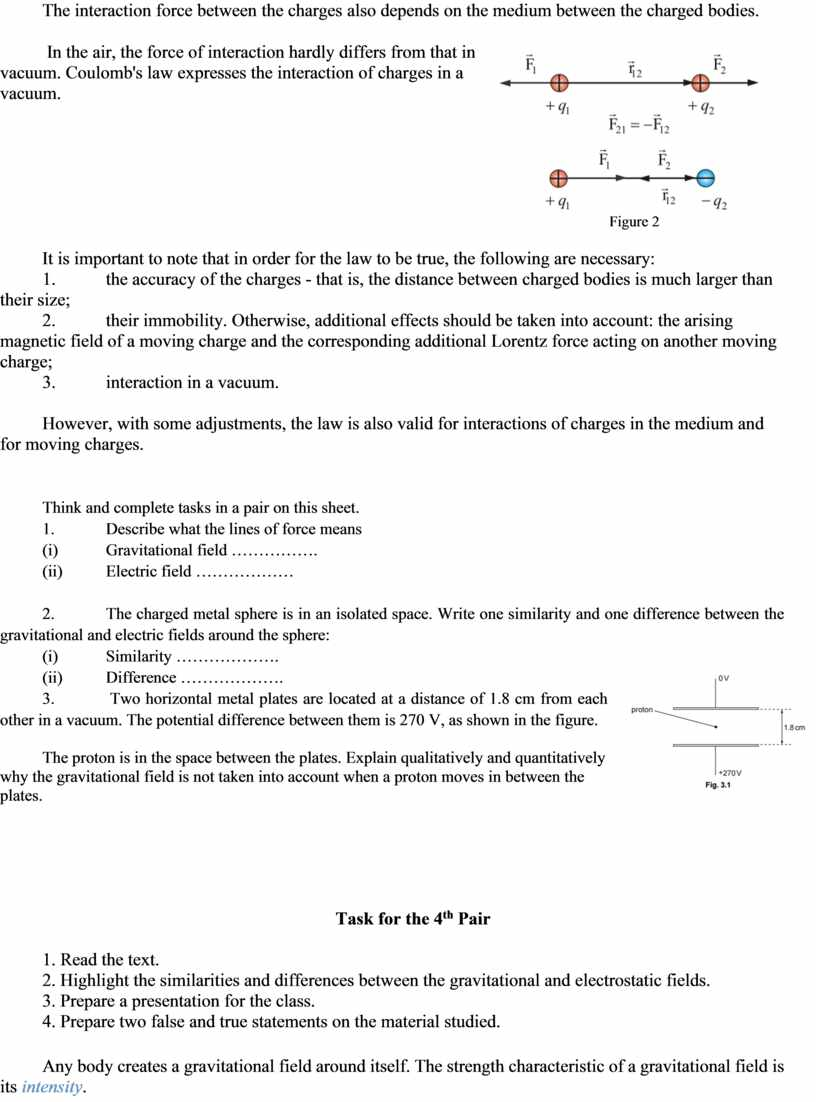## Let us need at some point in space to characterize the gravitational field created by a certain body of mass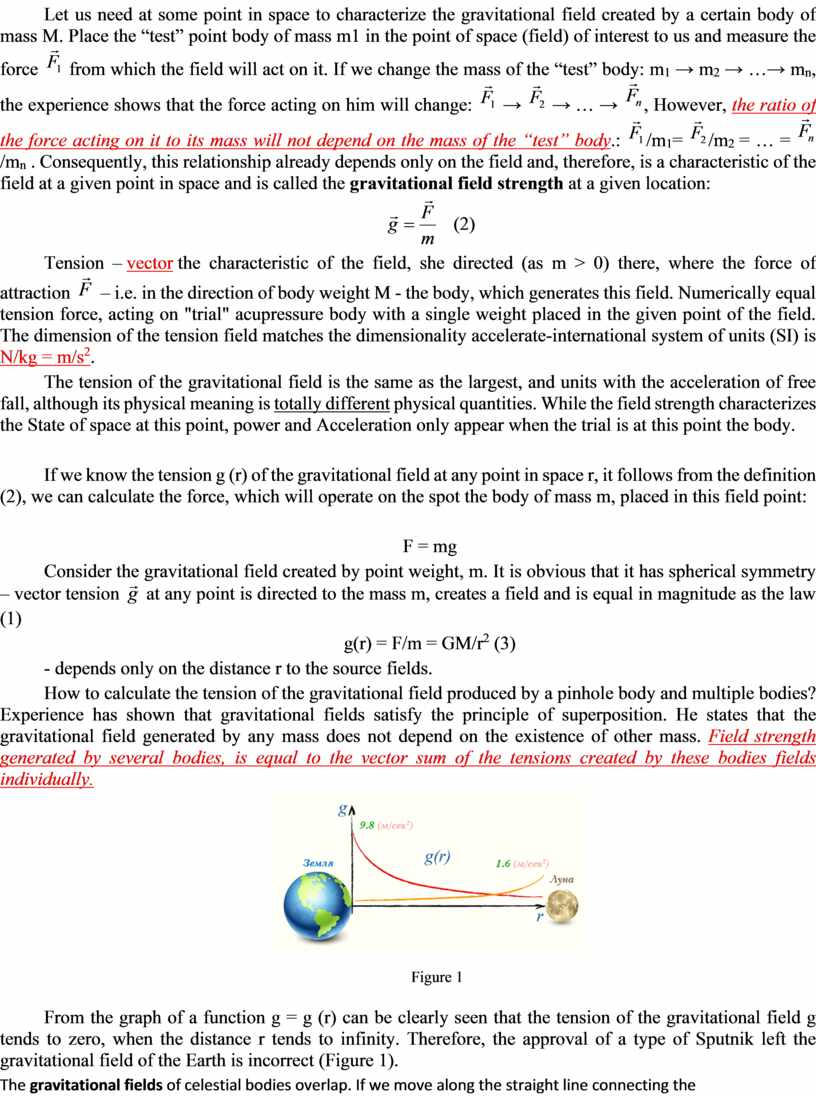## Earth and the Moon, then, starting from a certain place, the intensity of the gravitational field of the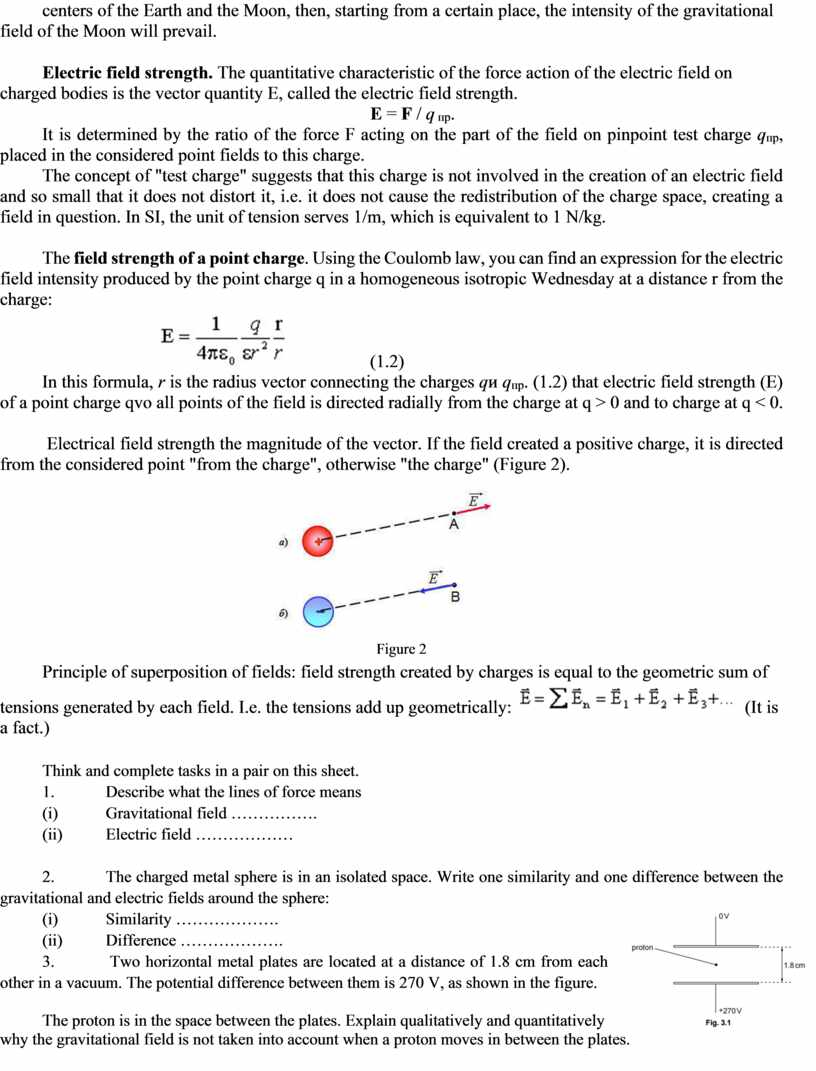## Equipotential surfaces of the gravitational field are shown with red lines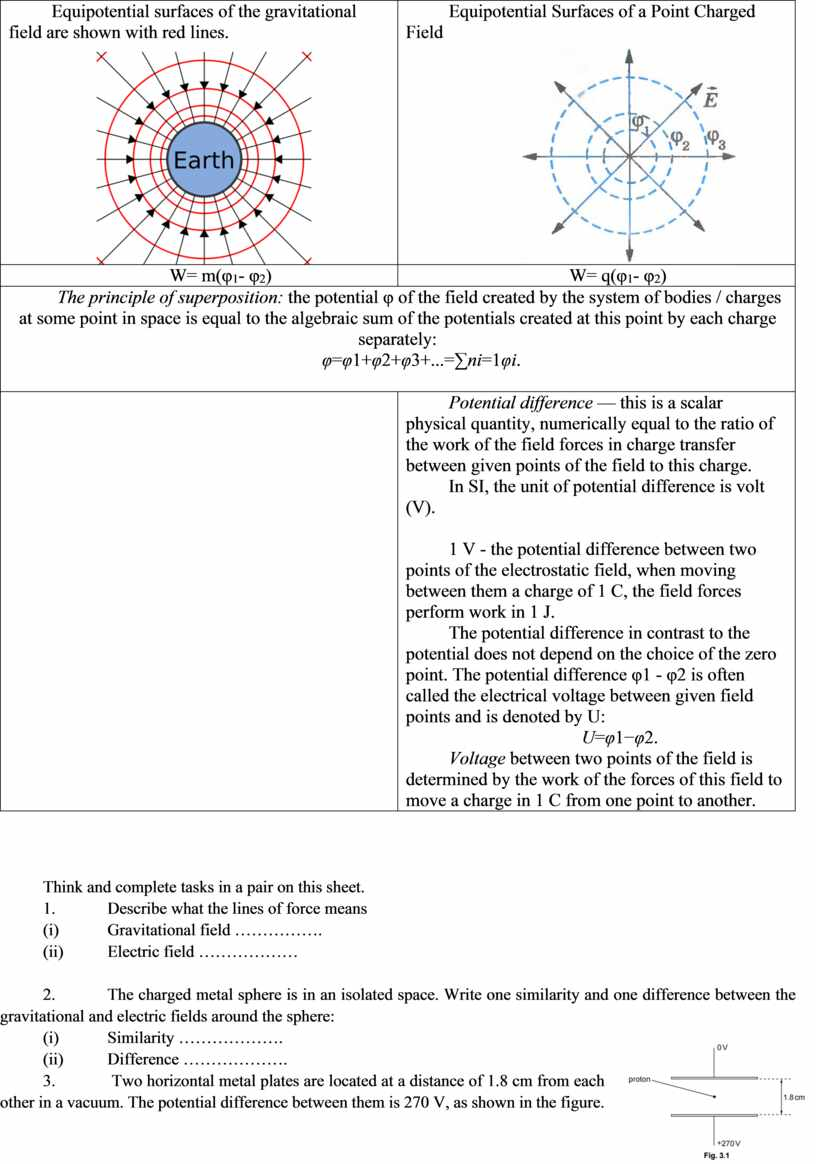Скачать файл